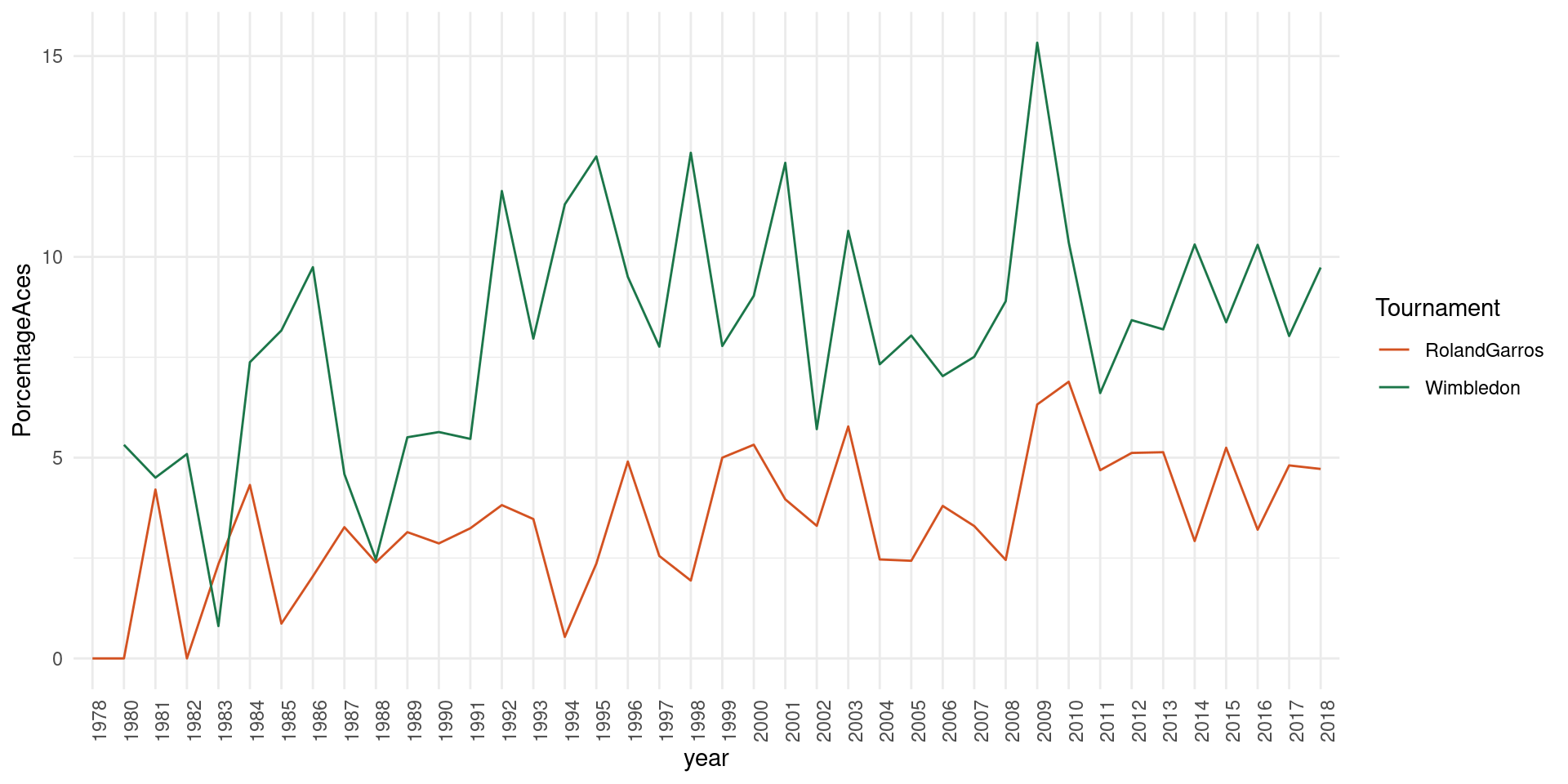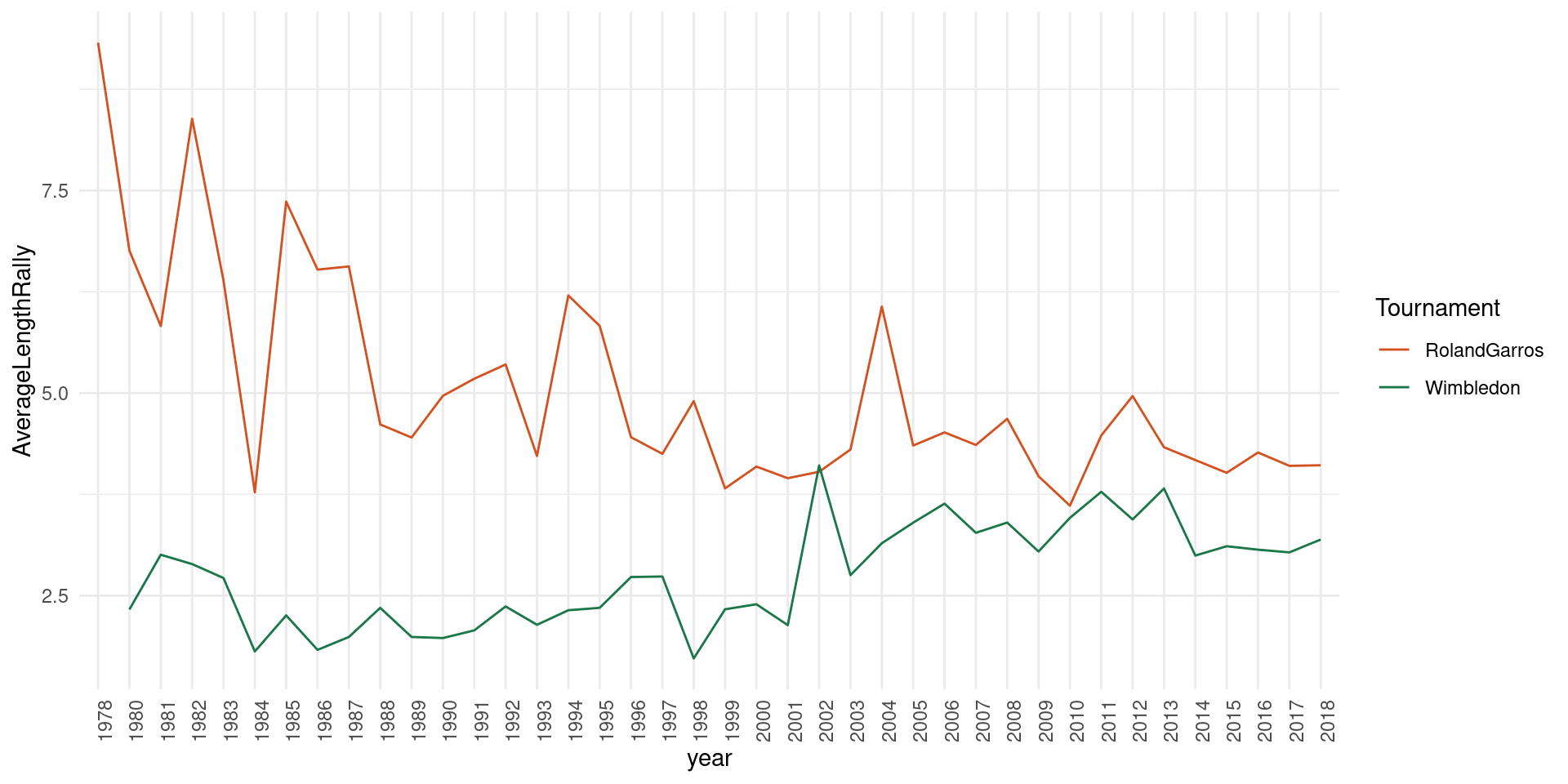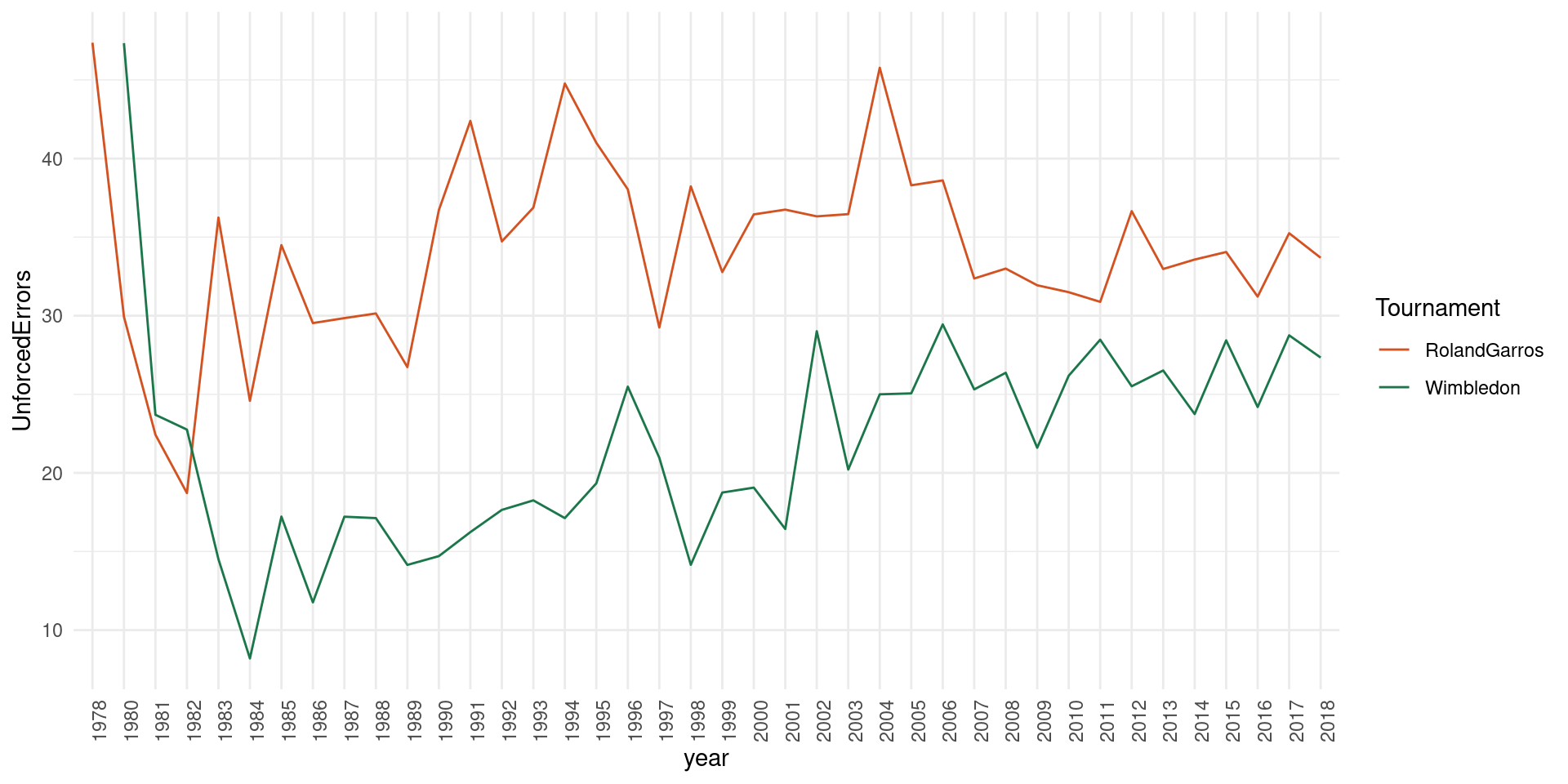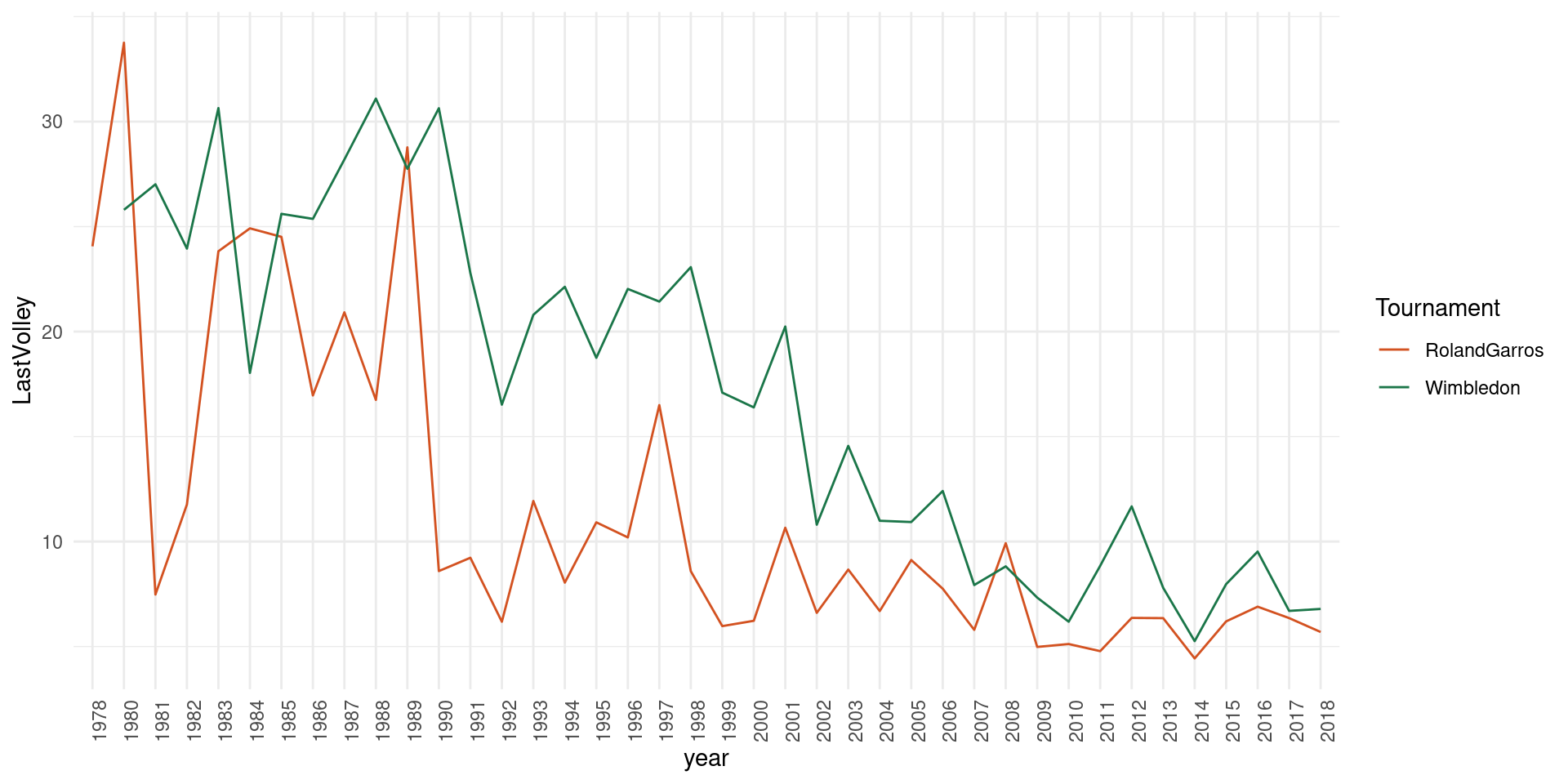# How tennis has changed over time

During the last years I have become a huge fan of tennis (both, on court and in TV). This year I followed (in TV) with attention Roland Garros and Wimbledon.

Although clay and grass are very different surfaces I’ve found the game very similar. What happened with the serve-volley game? I think it is my duty (as data freak) to found some data and play around with it.

To my delight I found The Match Charting Project by Jeff Sackman, standing ovation for this amazing project.

So, I’ve done some code to find out.

library(RCurl)

matches_file_m <- "https://raw.githubusercontent.com/JeffSackmann/tennis_MatchChartingProject/master/charting-m-matches.csv"
matches_file_w <- "https://raw.githubusercontent.com/JeffSackmann/tennis_MatchChartingProject/master/charting-w-matches.csv"
points_file_m <- "https://raw.githubusercontent.com/JeffSackmann/tennis_MatchChartingProject/master/charting-m-points.csv"
points_file_w <- "https://raw.githubusercontent.com/JeffSackmann/tennis_MatchChartingProject/master/charting-w-points.csv"

if(!exists("df_matches")){
df_matches <- bind_rows(matches_w,matches_m)
}
return(df_matches)
}

if(!exists("df_points")){
colnames(points_w) <- colnames(points_m)
points_m$TB. <- as.integer(points_m$TB.)
df_points <- bind_rows(points_w,points_m)
}
return(df_points)
}

get_players <- function(){
players <- unique(c(df_matches$Player.1,df_matches$Player.2))
return(players)
}

get_tournaments <- function(){
tournaments <- unique(df_matches$Tournament) return(tournaments) } cols <- c("RolandGarros" = "#D35221", "Wimbledon" = "#1B7649") df_points <- download_points() ## Warning in download_points(): NAs introduced by coercion df_matches <- download_matches() id_matches <- df_matches %>% dplyr::filter(Tournament %in% c("Roland Garros","Wimbledon")) %>% dplyr::select(match_id,Tournament) %>% dplyr::group_split(Tournament) l_point <- vector("list",2) for (i in 1:2){ l_point[[i]] <- df_points %>% dplyr::filter(match_id %in% id_matches[[i]]$match_id)
}
l_point[]$Tournament <- "RolandGarros" l_point[]$Tournament <- "Wimbledon"
l_point <- bind_rows(l_point)

#porcentaje de Aces
resultado1 <- l_point %>% dplyr::group_by(Tournament) %>% dplyr::summarise(PorcentajeAces=100*sum(isAce)/n())
resultado1
## # A tibble: 2 x 2
##   Tournament   PorcentajeAces
##   <chr>                 <dbl>
## 1 RolandGarros           4.03
## 2 Wimbledon              8.77
resultado2 <- l_point %>% dplyr::group_by(Tournament,year=substring(l_point$match_id,1,4)) %>% dplyr::summarise(PorcentageAces=100*sum(isAce)/n()) g1 <- ggplot(resultado2) + geom_line(aes(x=year,y=PorcentageAces,color=Tournament,group=Tournament)) + theme_minimal() + scale_colour_manual(values = cols) + theme(axis.text.x =element_text(angle = 90, hjust = 1)) plot(g1)Wimbledon still observes higher percentage of aces, but in both tournaments percentaje of aces has been growing. #longitud del punto resultado3 <- l_point %>% dplyr::group_by(Tournament) %>% dplyr::summarise(AverageLengthRally=mean(rallyLen)) resultado3 ## # A tibble: 2 x 2 ## Tournament AverageLengthRally ## <chr> <dbl> ## 1 RolandGarros 4.49 ## 2 Wimbledon 2.89 resultado4 <- l_point %>% dplyr::group_by(Tournament,year=substring(l_point$match_id,1,4)) %>% dplyr::summarise(AverageLengthRally=mean(rallyLen))

g2 <- ggplot(resultado4) + geom_line(aes(x=year,y=AverageLengthRally,color=Tournament,group=Tournament)) + theme_minimal() + scale_colour_manual(values = cols) + theme(axis.text.x =element_text(angle = 90, hjust = 1))
plot(g2)Here you have, Wimbledon still has shorter rallies but the difference is getting smaller. It has surprised me the length of the points in Roland Garros in the 70s and 80s.

#Errores no forzados
resultado5 <- l_point %>% dplyr::group_by(Tournament) %>% dplyr::summarise(UnforcedErrors=100*sum(isUnforced)/n())
resultado5
## # A tibble: 2 x 2
##   Tournament   UnforcedErrors
##   <chr>                 <dbl>
## 1 RolandGarros           33.9
## 2 Wimbledon              23.6
resultado6 <- l_point %>% dplyr::group_by(Tournament,year=substring(l_point$match_id,1,4)) %>% dplyr::summarise(UnforcedErrors=100*sum(isUnforced)/n()) g3 <- ggplot(resultado6) + geom_line(aes(x=year,y=UnforcedErrors,color=Tournament,group=Tournament)) + theme_minimal() + scale_colour_manual(values = cols) + theme(axis.text.x =element_text(angle = 90, hjust = 1)) plot(g3)Unforced errors in Wimbledon have been increasing, I guess this is related with the length of the rallies #Puntos que acaban en volea resultado7 <- l_point %>% dplyr::mutate(lastshot=str_sub(string = rallyNoDirection,start = -1)) %>% dplyr::mutate(lastisvolley=ifelse(lastshot %in% c("v","z","o","p"),TRUE,FALSE )) %>% dplyr::group_by(Tournament) %>% dplyr::summarise(LastVolley=100*sum(lastisvolley)/n()) resultado7 ## # A tibble: 2 x 2 ## Tournament LastVolley ## <chr> <dbl> ## 1 RolandGarros 8.37 ## 2 Wimbledon 13.3 resultado8 <- l_point %>% dplyr::mutate(lastshot=str_sub(string = rallyNoDirection,start = -1)) %>% dplyr::mutate(lastisvolley=ifelse(lastshot %in% c("v","z","o","p"),TRUE,FALSE )) %>% dplyr::group_by(Tournament,year=substring(l_point$match_id,1,4)) %>% dplyr::summarise(LastVolley=100*sum(lastisvolley)/n())

g4 <- ggplot(resultado8) + geom_line(aes(x=year,y=LastVolley,color=Tournament,group=Tournament)) + theme_minimal() + scale_colour_manual(values = cols) + theme(axis.text.x =element_text(angle = 90, hjust = 1))
plot(g4)This is striking, points finishing in volley have been decreasing abruptly and nowadays are difficult to see.

It seems that serve-volley game is outdated even in its sanctuary and baseline tennis reigns in Wimbledon